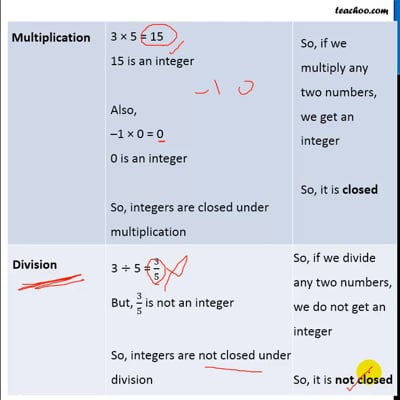Properties of Integers

Chapter 1 Class 7 Integers
Concept wise

For integers

Integers are both positive & negative numbers & zero

… −3, −2, −1, 0, 1, 2, 3,…..

 Operation Closed or not Addition 3 + 5 = 8 8 is an integer Also, –1 + 0 = –1 –1 is an integer So, integers are closed under addition So, if we add any two numbers, we get an integer So, it is closed Subtraction 3 – 5 = –2 –2 is an integer Also, –1 – 0 = –1 –1 is an integer So, integers are closed under subtraction So, if we subtract any two numbers, we get an integer So, it is closed Multiplication 3 × 5 = 15 15 is an integer Also, –1 × 0 = 0 0 is an integer So, integers are closed under multiplication So, if we multiply any two numbers, we get an integer So, it is closed Division 3 ÷ 5 = 3/5 But, 3/5 is not an integer So, integers are not closed under division So, if we divide any two numbers, we do not get an integer So, it is not closedThis video is only available for Teachoo black users

Introducing your new favourite teacher - Teachoo Black, at only ₹83 per month